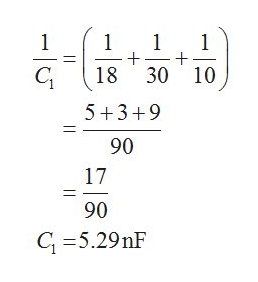# Part B through C Please. For the system of capacitors shown in the figure below(Figure 1), a potential difference of 25.0 V is maintained across ab.Part B How much charge is stored by this system?Part C How much charge does the 6.50 nF capacitor store?

Question
157 views

For the system of capacitors shown in the figure below(Figure 1), a potential difference of 25.0 V is maintained across ab.

Part B
How much charge is stored by this system?
Part C
How much charge does the 6.50 nF capacitor store?

check_circle

Step 1

For part B, First calculate the equivalent capacitance of the System,

Solve for the series capacitances in the above circuit as,help_outlineImage Transcriptionclose1 1 1 + 30 10 1 18 5+3+9 90 17 90 C 5.29nF || fullscreen
Step 2

Now calculate the Equivalent capacitance Ceq as ,

Step 3

The expression for the total charge stored by this system is given by,

Qtotal = CeqV

Substitute 1...

### Want to see the full answer?

See Solution

#### Want to see this answer and more?

Solutions are written by subject experts who are available 24/7. Questions are typically answered within 1 hour.*

See Solution
*Response times may vary by subject and question.
Tagged in

### Electrostatic Potential and Capacitance